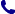• Kontakt
• Newsletter Zapisz się po informacje o nowościach i specjalnych ofertach. Administrator danych: ABE-IPS sp. z o.o.
Wybierz interesujące Cię kategorie
Wyrażam zgodę na przetwarzanie moich danych osobowych w celach marketingowych, tym samym akceptuję Regulamin Newslettera i Politykę prywatności.
Wyrażam zgodę na przesyłanie informacji handlowych drogą elektroniczną.
Wypisz się
• Klienci instytucjonalni
• Dostawa
• O nas
Dostęp on-line

# Książki

0.00 PLN
Schowek (0)
 Schowek jest pusty

## Topology, Calculus and Approximation

 Autorzy Wydawnictwo Springer Nature Customer Service Center GmbH Data wydania 01/04/2017 Wydanie Pierwsze Liczba stron 382 Forma publikacji książka w miękkiej oprawie Poziom zaawansowania Dla szkół wyższych i kształcenia podyplomowego Język angielski ISBN 9781447173151 Kategorie Rachunek różniczkowy i formuły, Analiza numeryczna
230.12 PLN (z VAT)
\$51.76 / £42.83 / €49.34 /
Produkt na zamówienie
Dostawa 5-6 tygodni
Ilość
Do schowka

## Opis książki

Presenting basic results of topology, calculus of several variables, and approximation theory which are rarely treated in a single volume, this textbook includes several beautiful, but almost forgotten, classical theorems of Descartes, Erdos, Fejer, Stieltjes, and Turan. The exposition style of Topology, Calculus and Approximation follows the Hungarian mathematical tradition of Paul Erdos and others. In the first part, the classical results of Alexandroff, Cantor, Hausdorff, Helly, Peano, Radon, Tietze and Urysohn illustrate the theories of metric, topological and normed spaces. Following this, the general framework of normed spaces and Caratheodory's definition of the derivative are shown to simplify the statement and proof of various theorems in calculus and ordinary differential equations. The third and final part is devoted to interpolation, orthogonal polynomials, numerical integration, asymptotic expansions and the numerical solution of algebraic and differential equations. Students of both pure and applied mathematics, as well as physics and engineering should find this textbook useful. Only basic results of one-variable calculus and linear algebra are used, and simple yet pertinent examples and exercises illustrate the usefulness of most theorems. Many of these examples are new or difficult to locate in the literature, and so the original sources of most notions and results are given to help readers understand the development of the field.

Topology, Calculus and Approximation

## Spis treści

Part 1. Topology.- Chapter 1. Metric spaces.- Chapter 2. Topological spaces.- Chapter 3. Normed spaces.- Part 2. Differential calculus.- Chapter 4. The Derivative.- Chapter 5. Higher-order derivatives.- Chapter 6. Ordinary differential equations.- Chapter 7. Implicit functions and their applications.- Part 3. Approximation methods.- Chapter 8. Interpolation.- Chapter 9. Orthogonal polynomials.- Chapter 10. Numerical integration.- Chapter 11. Finding roots.- Chapter 12. Numerical solution of differential equations.

## Polecamy również książki801 777 223